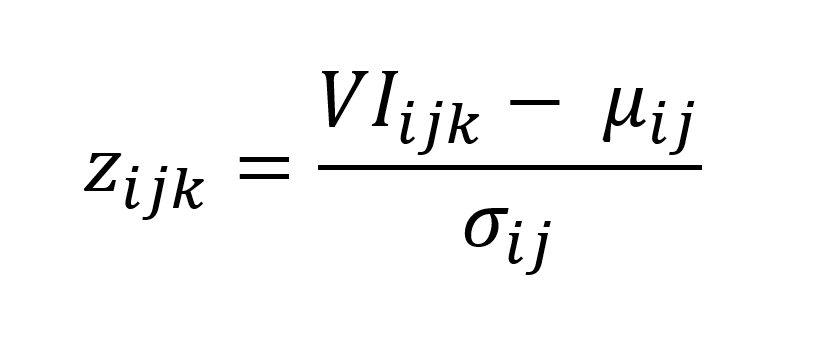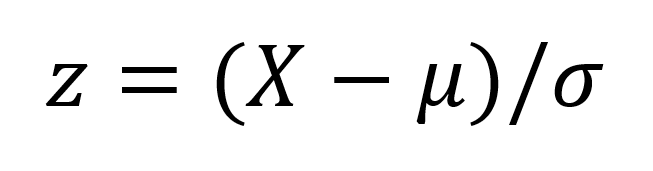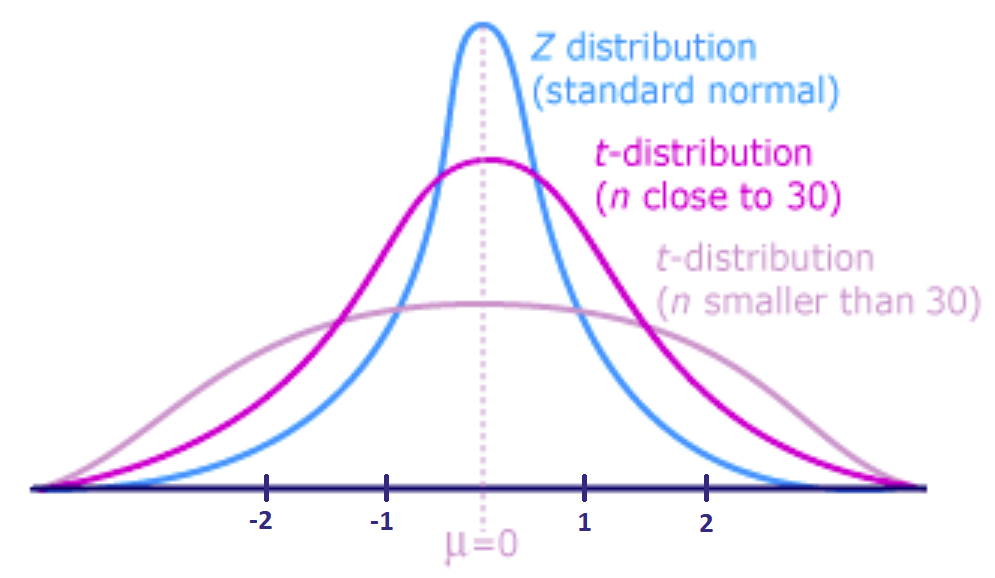# Standardized Vegetation Index (SVI)

The Standardized Vegetation Index (SVI) provides information about the relative vegetation condition compared to the years being analyzed. As the state of vegetation is influenced by weather conditions the SVI can also be utilized as a drought-index and for regular drought monitoring to facilitate the early identification of the slow-onset natural hazard. The SVI is based on satellite Vegetation Indices (VI) such as the Normalized Difference Vegetation Index (NDVI) or Enhanced Vegetation Index (EVI) indicating the estimated vegetation condition. Vegetation Indices mostly explore the difference of reflectance radiated in the visible red and near infrared waveband for determining the health or stress of plants as well as the density of plant growth.

The key strengths and limitations of the SVI are outlined below

## Definition of the SVI

Originally building on the NDVI anomaly concept, the Standardized Vegetation Index (SVI) developed by Peters et al. (2002) describes the probability of variation from the normal NDVI over multiple years of data (e.g., 12 years), on a weekly time step. The SVI is a z-score deviation from the mean in units of the standard deviation, calculated from the NDVI or EVI values for each pixel location of a composite period for each year during a given reference period. The equation below shows the general calculation of the SVIwhere zijk is the z-value for the pixel i during week j for year k, VIij is the weekly VI value for pixel i during week j for year k whereby both the NDVI or EVI can be utilized as VI, µij is the mean for pixel i during week j over n years, and σij is the standard deviation of pixel i during week j over n years.

## Standard normal distribution

The normal random variable of a standard normal distribution is called a standard score or a Z-score. Every random variable X can be transformed into a Z-score via the following equation:where X is a normal random variable, μ is the mean, and σ is the standard deviation. Consequently,

• a z-score equal to 0 represents an element equal to the mean,
• a z-score less than 0 represents an element less than the mean, and
• a z-score greater than 0 represents an element greater than the mean.

The Z-score indicates how many standard deviations an element is away from the mean whereby the standard deviation, in general, indicates how spread out the set of data is. A low standard deviation implies that the data is closely clustered around the mean whereas a high standard deviation implies that the data is dispersed over a wider range of values.
If the number of elements in the dataset is large, about 68% of the data fall within 1 standard deviation of the mean, 95% within 2 standard deviations and 99.7% within 3 standard deviations of the mean.(Source)

## Standard normal distribution and t-distribution

By means of the Z-score, one can decide if the sample being analyzed differs from the population mean. The Z distribution is a standard normal distribution and can be used if the following terms are known and given:

• the population mean,
• the population standard deviation,
• the sample mean, and
• the sample size above 30.

However, if the population standard deviation is not known and if the sample size is less than 30 (a rule of thumb which is not strict), the use of the Z value is inappropriate. The T-score which resembles the Z-score should be used instead.The following graph illustrates the Z distribution which is the standard normal distribution and the t-distribution which is characterized by a lower mean average and thicker tails compared to the Z distribution.(Source)

When using the SVI for relatively comparing the vegetation condition per year, a single year should be considered as one sample. Therefore, if the available or selected time period of analyzed satellite images is less than 30 years, a t-distribution table should be used for further determining the confidence level of the SVI outcome. Otherwise, a standard normal table or Z table should be used.

## Key Strengths

• The SVI provides a useful analysis tool for the assessment of vegetation condition trends and monitoring of areas affected by drought as it relatively compares the vegetation condition of a point of time of a year with the respective points of time in the other years included in the analysis. The user can, therefore, estimate whether the state of the vegetation in the period of interest is unusually good or bad compared to other years.
• The SVI allows a clear visualization of relative vegetation greenness at each pixel location. It indicates the deviations of the present vegetation condition from the mean vegetation condition in a specific pixel deriving from the z-score as outlined above.
• The computation of the SVI is not restricted to the commonly utilized NDVI which makes the SVI more variable. Depending on the area of interest, the user can further derive the SVI from other Vegetation Indices, such as the Enhanced Vegetation Index (EVI) with an improved sensitivity over dense vegetation conditions compared to the NDVI.
• The temporal and spatial coverage of the SVI for example for regular drought monitoring depends on both the underlying vegetation index and the satellite data used. For example, the NDVI values can be acquired by the Advanced Very High-Resolution Radiometer (AVHRR) sensor with a resolution of 1.1 km and a total global coverage twice a week. Next, to this fast temporal ability, the NDVI values can be analyzed since the launch of the AVHRR in 1981 allowing a relative comparison of the vegetation condition over almost 40 years by means of the SVI.

## Key Limitations

• The Vegetation Indices, on which the SVI is based, do not differentiate between different types and characteristics of vegetation. The SVI can only provide a relative comparison of the vegetation condition while the assessed deviation from the mean vegetation condition cannot be translated into an absolute deviation of for example the plant height. Neither can the SVI be interpreted for an absolute quantification of agricultural damage.
• The SVI is a good indicator of vegetation response to short-term weather condition. However, if interpreted as a drought index it must be considered that other climatic, hydrologic or agricultural conditions apart from drought can also cause reduced vegetation vigour. Relatively poor vegetation condition may further be induced by flooding, unseasonable coolness, or crop rotation. Therefore, to better interpret the vegetation trend in a specific region, ground data must also be included in the analysis.
• The SVI measures the vegetation response to drought hazard, but it cannot be used to quantify the magnitude of  a drought hazard
• Anyamba, A. and Tucker, C.J., 2012. Historical perspective of AVHRR NDVI and vegetation drought monitoring. Remote Sens Drought: Innovative Monit Approaches, 23